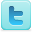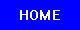﻿ Electrical Resistivity Experiments for Lesson Plans & Science Fair Projects
Electrical Resistivity
K-12 Experiments & Background Information
For Science Labs, Lesson Plans, Class Activities, Homework Help & Science Fair Projects
For Middle and High School Students and Teachers

Resistivity Experiments

Resistivity Background Information

## Definitions

### Resistivity vs. Resistance

Electrical resistivity is a measure of how strongly a standard volume of material opposes the flow of electric current.

Electrical resistance is a measure of how strongly a bulk of material opposes the flow of electric current.

## Basics

Electrical resistivity (also known as specific electrical resistance) is a measure of how strongly a material opposes the flow of electric current. A low resistivity indicates a material that readily allows the movement of electrical charge. The SI unit of electrical resistivity is the ohm metre.

The electrical resistivity ρ (rho) of a material is given by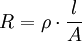where

ρ is the static resistivity (measured in ohm-metres, Ωm);
R is the electrical resistance of a uniform specimen of the material (measured in ohms, Ω);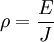where

E is the magnitude of the electric field (measured in volts per metre, V/m);
J is the magnitude of the current density (measured in amperes per square metre, A/m²).

Finally, electrical resistivity is also defined as the inverse of the conductivity σ (sigma), of the material, orThe resistance of a given sample will increase with the length, but decrease with the cross sectional area. If one uses the common hydraulic analogy, pushing 5 gallons per minute through a garden hose would meet with some resistance. 5 gpm through a 4" diameter pipe would be relatively easy, requiring less pressure. Cross sectional area reduces resistance. Similarly, a 12 ft garden hose will fill a 5 gallon bucket in a certain amount of time. Filling the same bucket from the same spigot but with 500 feet of hose will fill the bucket in a significantly longer time. Resistance increases with length.

Resistance is measured in ohms. Length over Area has units of 1/distance. To end up with ohms, resistivity must be in the units of "ohms × distance" (SI ohm-metre, US ohm-inch).

## Table of resistivities

This table shows the resistivity and temperature coefficient of various materials. The values are correct at 20 °C (68 °F)

Material Resistivity (Ωm) Coefficient*
Silver 1.59×10−8 .0038
Copper 1.7×10−8 .0039
Gold 2.44×10−8 .0034
Aluminium 2.82×10−8 .0039
Tungsten 5.6×10−8 .0045
Brass 0.8×10−7 .0015
Iron 1.0×10−7 .005
Platinum 1.1×10−7 .00392
Manganin 4.82×10−7 .000002
Constantan 4.9×10−7 .
Mercury 9.8×10−7 .0009
Nichrome 1.10×10−6 .0004
Carbon 3.5×10−5 -.0005
Germanium 4.6×10−1 -.048
Silicon 6.40×102 -.075
Glass 1010 to 1014 ?
Hard rubber approx. 1013 ?
Sulfur 1015 ?
Paraffin 1017 ?
Quartz (fused) 7.5×1017 ?
PET 1020 ?
Teflon 1022 to 1024 ?

*The numbers in this column increase or decrease the significand portion of the resistivity. For example, at 21°C (294.15 K), the resistivity of silver is 1.65×10−8.

## Temperature dependence

In general, electrical resistivity of metals increases with temperature, while the resistivity of semiconductors decreases with increasing temperature. In both cases, electron-phonon interactions can play a key role. At high temperatures, the resistance of a metal increases linearly with temperature. As the temperature of a metal is reduced, the temperature dependence of resistivity follows a power law function of temperature. Mathematically the temperature dependence of the resistivity ρ of a metal is given by the Bloch-Gruneissen formula :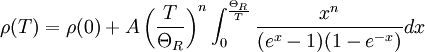$\rho(T)=\rho(0)+A\left(\frac{T}{\Theta_R}\right)^n\int_0^{\frac{\Theta_R}{T}}\frac{x^n}{(e^x-1)(1-e^{-x})}dx$

where ρ(0) is the residual resistivity due to defect scattering, A is a constant that depends on the velocity of electrons at the fermi surface, the Debye radius and the number density of electrons in the metal. ΘR is the Debye temperature as obtained from resistivity measurements and matches very closely with the values of Debye temperature obtained from specific heat measurements. n is an integer that depends upon the nature of interaction:

1. n=5 implies that the resistance is due to scattering of electrons by phonons (as it is for simple metals)
2. n=3 implies that the resistance is due to s-d electron scattering (as is the case for transition metals)
3. n=2 implies that the resistance is due to electron-electron interaction.

As the temperature of the metal is sufficiently reduced (so as to 'freeze' all the phonons), the resistivity usually reaches a constant value, known as the residual resistivity. This value depends not only on the type of metal, but on its purity and thermal history. The value of the residual resistivity of a metal is decided by its impurity concentration. Some materials lose all electrical resistivity at sufficiently low temperatures, due to an effect known as superconductivity.

An even better approximation of the temperature dependence of the resistivity of a semiconductor is given by the Steinhart-Hart equation: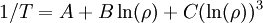$1/T = A + B \ln(\rho) + C (\ln(\rho))^3 \,$

where A, B and C are the so-called Steinhart-Hart coefficients.

This equation is used to calibrate thermistors.

## Complex resistivity

When analysing the response of materials to alternating electric fields, as is done in certain types of tomography, it is necessary to replace resistivity with a complex quantity called impeditivity, in analogy to electrical impedance. Impeditivity is the sum of a real component, the resistivity, and an imaginary component, the reactivity (reactance) .

## Resistivity density products

In some applications where the weight of an item is very important resistivity density products are more important than absolute low resistivity- it is often possible to make the conductor thicker to make up for a higher resistivity; and then a low resistivity density product material (or equivalently a high conductance to density ratio) is desirable. For example, for long distance overhead power lines— aluminium is frequently used rather than copper because it is lighter for the same conductance.

In practice, calcium and the alkali metals are rarely used for conductors due to their high reactivity with water and oxygen.

Source: Wikipedia (All text is available under the terms of the GNU Free Documentation License and Creative Commons Attribution-ShareAlike License.)

Science Fair Projects Resources [Resource]
Electrical Safety [Resource] [Resource]
Electricity Science Fair Projects Books